Select Page

Common Core Standard: 8.F.A.2, 8.F.A.3

Use: Individually as a quick Sticky Math Warmup, in groups of three for a longer Sticky Math Warmup, or all together as a Sticky Math Lesson

Note: Post It sells sticky notes with grids on them, which makes using Sticky Math with graphing much simpler.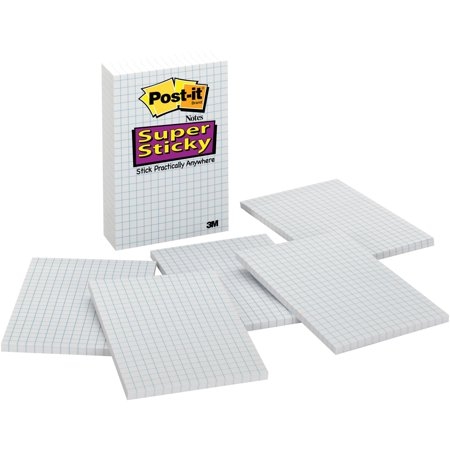STAGE ONE: EXPLORATION OF SLOPE-INTERCEPT FORM

The Sticky Math Pair below allows for the discovery of the slope and the intercept in two different forms.  Care should be made to elicit, use, and connect various vocabulary like rate of change, slope, rise-over-run, y-intercept, and constant.Connections could include:

• to move from (0,1) to (3,3)  you move vertically (rise) two units and move horizontally (run) three units
• the line crosses the y axis at (0,1)
• one way to graph a line might be to begin at (0,1), the y-intercept, then add points by moving vertically two units and horizontally three units
• if you make x=0 then you get 1, which is the point (0,1) or the y-intercept
• if you move vertically two units and horizontally three units from any point, then you are back on the line

The blank sticky is a reminder that students could create their own representations; these might include:

• a t-chart or table of values
• a context for the problem like, “I started with 1 dollar.  I got 2 dollars every 3 days.”

Creating a verbal description can be a fantastic formative assessment and lead to a higher DOK level.  You might give an example the first time if no student creates one of their own using the line, “someone in another class wrote this…” You don’t have to tell them the person in the other class was you!  Later, you might ask for verbal descriptions as one of their alternate representations as a formative assessment of their conceptual understanding.  Hopefully, at some point, students would be comfortable creating them on their own and just do it by themselves. Creating different contexts could also make the task more culturally relevant.  If students struggle in this area or as introduction, then create Sticky Math Pairs or a Sticky Math Triad like the one below: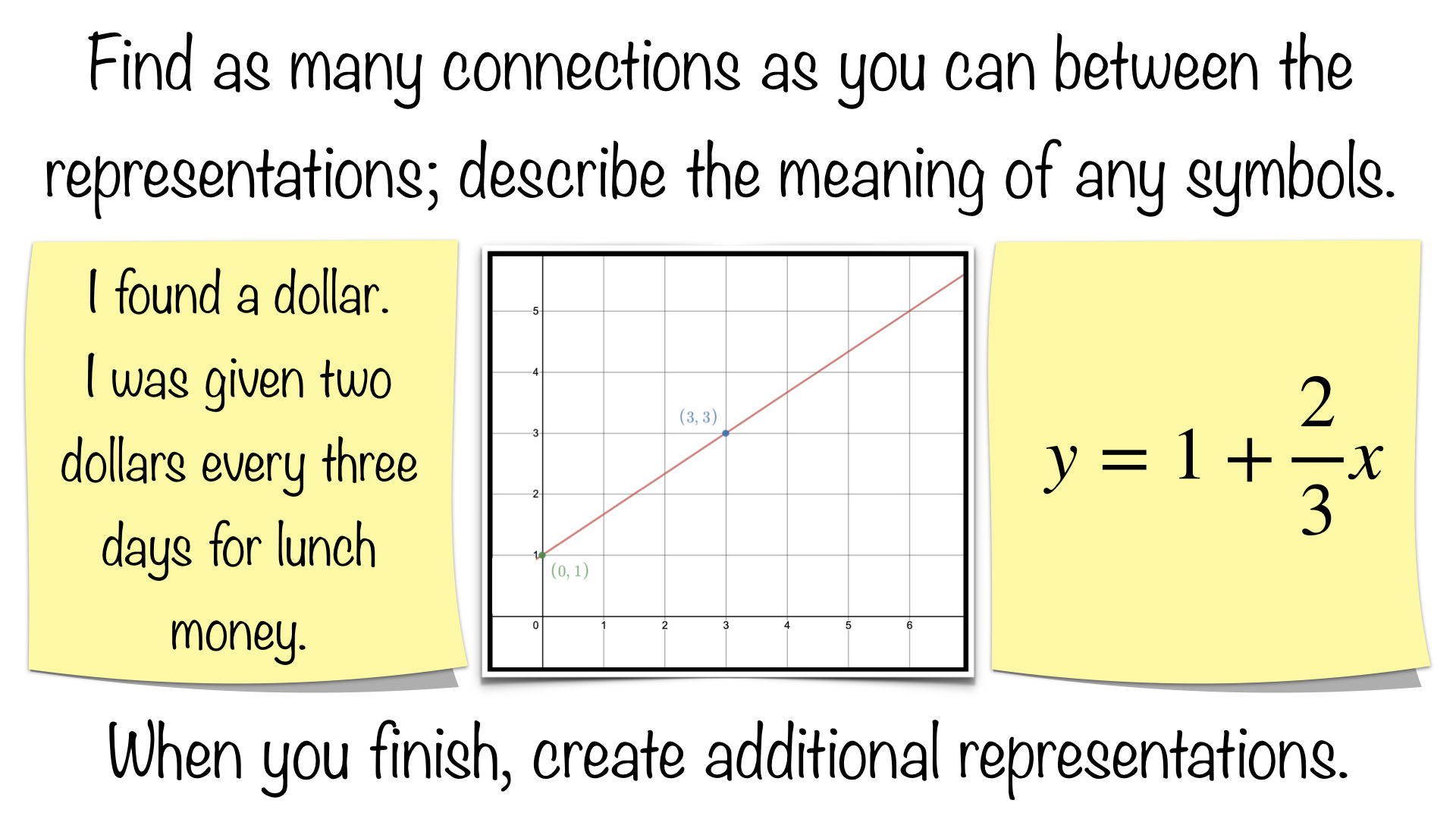Notice the equation is written with the constant first to match the verbal description chronologically and to change it up in order to help students achieve procedural fluency.

STAGE TWO: EXPLORATION OF THE Y-INTERCEPT

If you are doing one Sticky Math Pair per day as a shorter Sticky Math warmup, then you might move to the second Sticky Math Pair below followed by the Sticky Math Comparison on the third day.  The three of them are together here to show how you could group them into a slightly longer Sticky Math Warmup in order to make the point about the meaning of the y-intercept more explicitly.  Alternatively, you could just jump to the Sticky Math Comparison to accomplish the same thing more quickly.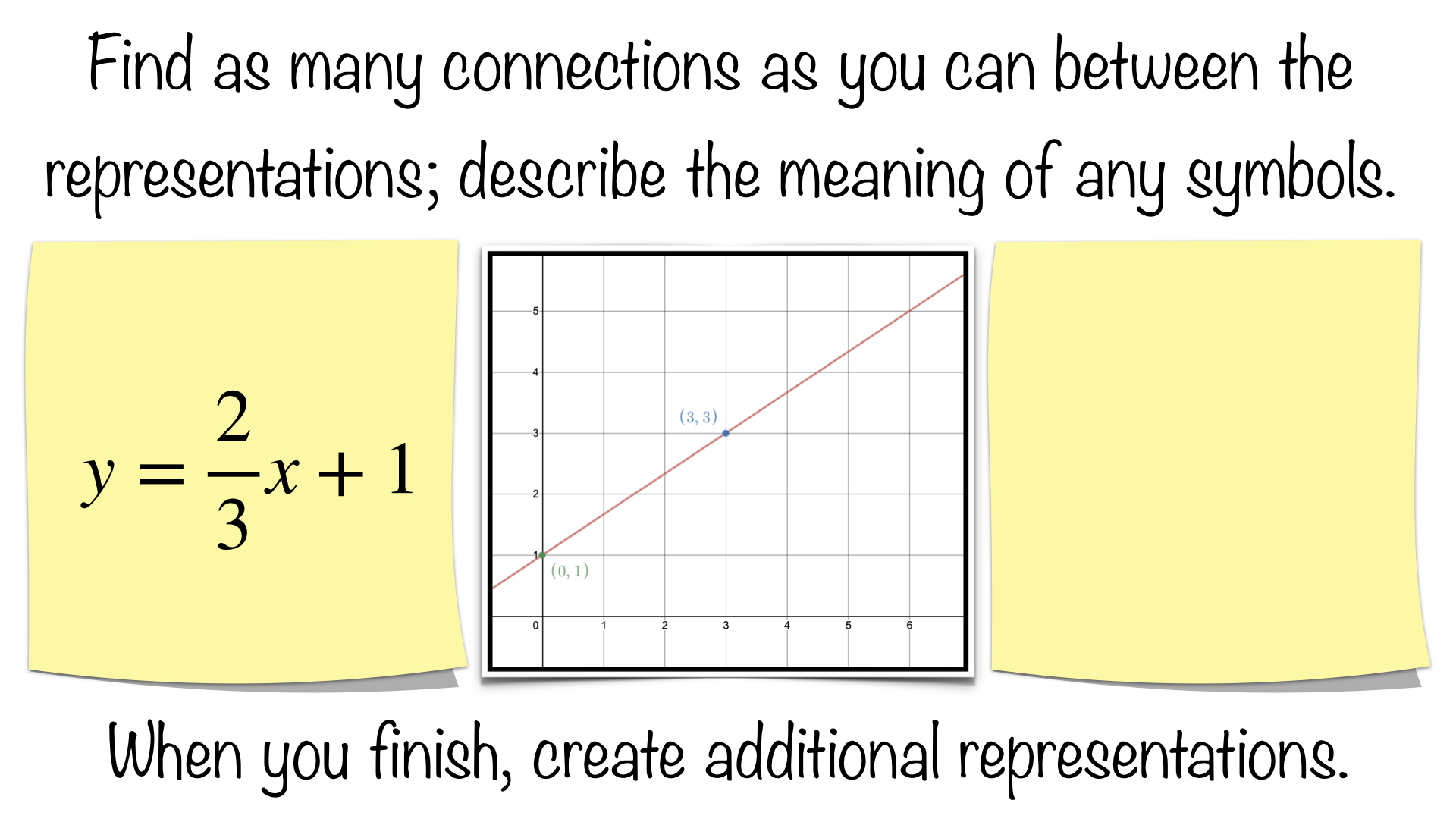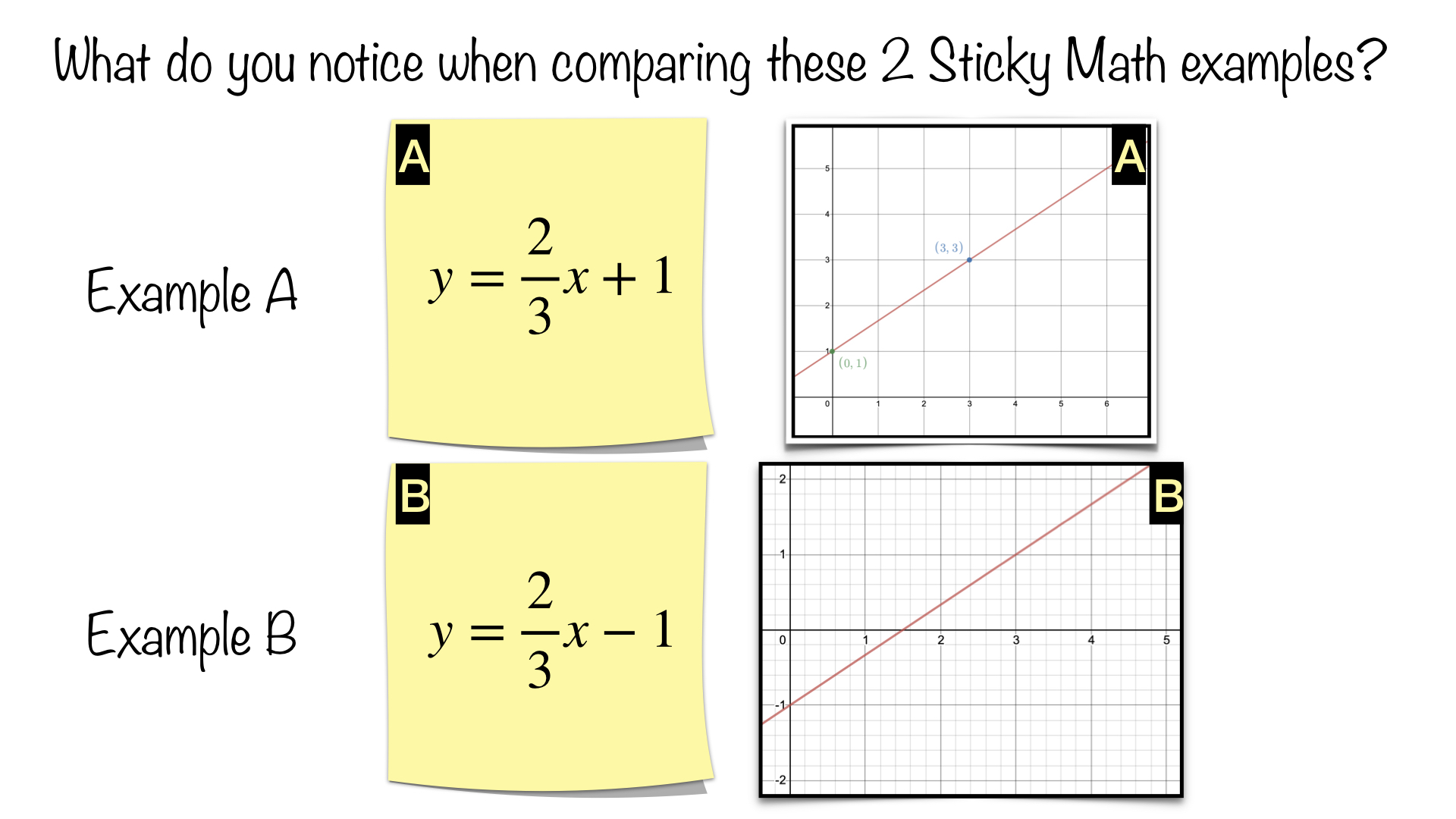Connections could include:

• any connection from stage one
• in Example A the line goes through (0,1), but in example B the line goes through (0,-1)
• the slopes are the same in both
• the intercepts are opposites of one another on a number line or y-axis, negative means opposite
• the grids on the graphs are different, the smaller boxes on the lower graph show a change of .2

The blank sticky is a reminder that students could create their own representations; these might include:

• any representation from stage one
• a new t-chart for Example B
• a context for Example B like, “I started in debt 1 dollar.  I got two dollars every three days.”

STAGE THREE: EXPLORATION OF SLOPE

If you are doing one Sticky Math Pair per day as a shorter Sticky Math warmup, then you might move to the second Sticky Math Pair below followed by the Sticky Math Comparison on the third day.  The three of them are together here to show how you could group them into a slightly longer Sticky Math Warmup in order to make the point about the meaning of the negative slope more explicitly.  Alternatively, you could just jump to the Sticky Math Comparison to accomplish the same thing more quickly.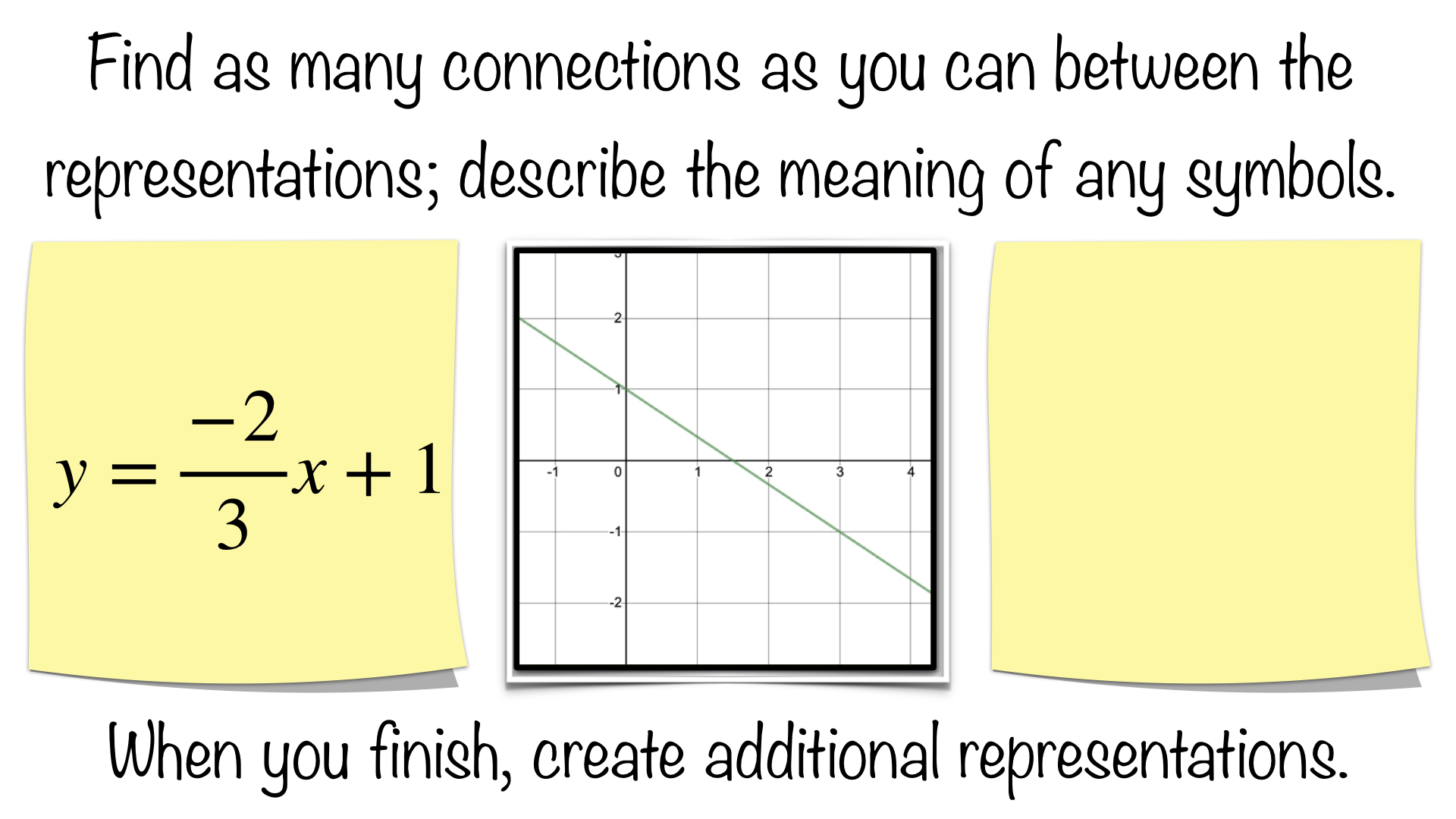Connections could include:

• any thing from stage one or two
• the intercepts are the same
• the negative makes the line “go down” as you read it like a book from left to right
• they both move horizontally 3 (run)
• they move in the opposite direction vertically by two (rise), negative means opposite

Students could create their own representations; these might include:

• anything from stage one or two
• a t-chart or table of values for Example B
• a verbal description like, “I started with 1 dollar.  A bully told me I owed him 2 dollars every 3 days. I have not been to school since!”

TO BE CONTINUED…

This topic is further explored in the post Negatives in a Slope Fraction.  Sticky Math Comparisons and Sticky Math Matching activities are included there.

Common Core Standards: 8.F.A.2, 8.F.A.3

What connections are your students making?  What modifications are you making to use this with students?  We would love to hear your feedback; please submit a comment below or consider submitting your own Sticky Math activity here.